Courses

# Dual Nature Of Matter and Uncertainty Principle Class 11 Notes | EduRev

## Class 11 : Dual Nature Of Matter and Uncertainty Principle Class 11 Notes | EduRev

The document Dual Nature Of Matter and Uncertainty Principle Class 11 Notes | EduRev is a part of the Class 11 Course Chemistry Class 11.
All you need of Class 11 at this link: Class 11

DeBroglie's hypothesis:

DeBroglie proposed that like light, electron possess dual nature i.e. behave like both as material particle and as wave. The concept of dual character of metal evolved a wave mechanical theory of atom according to which electrons. protons and even atoms possess wave properties when moving.Fig: De Broglie Wavelength Hypothesis.

Derivation of De Broglie's Relationship :

For a photon,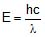...(i)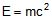...(ii)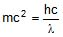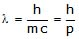He concluded that as electromagnetic radiation have some associated mass or associated momentum, in the same way every moving particle of mass `m' and velocity `v' is associated with waves and these associated waves are known as matter waves or De Broglie's waves.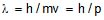Experimental verification of De Broglie's hypothesis : Given by Davission and Germer.

They observed that a beam of electrons are diffracted by nickel crystal just like the x-rays. More over the wavelength of the electrons determined by the diffraction experiments are equal to the values calculated from De Broglie's relationship.

Derivation of Bohr's postulate of quantization of angular momentum from De Broglie's Equations-

According to De Broglie, moving electron is associated with waves must be completely in phase and therefore, only those orbits are possible where circumference of the orbit is integral multiple of λ i.e.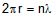, where n is the no. of waves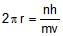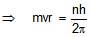From the above expression it can be commented that

no of waves in a shell waves = shell no.
Calculation of De Broglie wavelength if K.E. of the particle is E :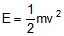2E = mv2; 2mE = m2 v= p2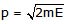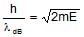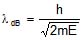If a charge particle at rest (having charge `q') is accelerated by potential difference'V' volt then,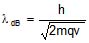,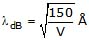(Only for electron).

Ex. Calculate λdB of electron having K.E. 3 eV.

Sol.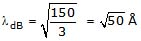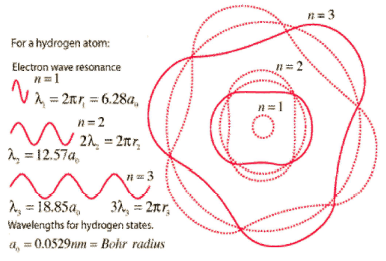Heisenberg Uncertainty Principle: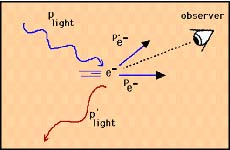Fig: Uncertainty Principle.

The position and momentum of a particle cannot be simultaneously measured with arbitrarily high precision. There is a minimum for the product of the uncertainties of these two measurements. There is likewise a minimum for the product of the uncertainties of the energy and time.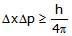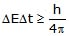The uncertainty principle contains implications about the energy that would be required to contain a particle within a given volume. The energy required to contain particles comes from the fundamental forces, and in particular the electromagnetic force provides the attraction necessary to contain electrons within the atom, and the strong nuclear force provides the attraction necessary to contain particles within the nucleus. But Planck's constant, appearing in the uncertainty principle, determines the size of the confinement that can be produced by these forces. Another way of saying it is that the strengths of the nuclear and electromagnetic forces along with the constraint embodied in the value of Planck's constant determine the scales of the atom and the nucleus.

Wave mechanical model of an Atom:

Schrodinger equation:

The Schrodinger equation plays the role of Newton's laws and conservation of energy in classical mechanics - i.e., it predicts the future behavior of a dynamic system. It is a wave equation in terms of the wave function which predicts analytically and precisely the probability of events or outcome.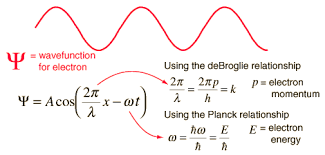Fig: Schrodinger wave equation.The detailed outcome is not strictly determined, but given a large number of events, the Schrodinger equation will predict the distribution of results.

Hy = EY

The kinetic and potential energies are transformed into the Hamiltonian which acts upon the wave function to generate the evolution of the wave function in time and space. The Schrodinger equation gives the quantized energies of the system and gives the form of the wave function so that other properties may be calculated.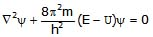Where , E = total energy of electron

U = potential energy of electron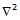= Laplacian operator =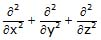and y = wave function of electron = amplitude of matter wave.

Schordinger equation can be solved completely for hydrogen atom and hydrogen-like species like He , Li.

The Schordinger equation can also be written in terms of spherical polar coordinates (r, q, f) in addition to Cartesian coordinate x, y, z.

Since, H-atom possess spherical symmetry, it is easier to solve Schrodinger equation if it is represented in polar coordinate.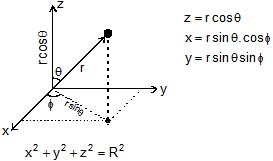When Schrodinger equation in polar coordinate is solved for H-atom then the solution obtained can be expressed as: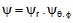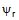represents radial wave function which depends on n, l.

→ Angular wave function which depends on m, l.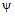(Psi) : [Wave function or Amplitude of Electron Wave]

It is also known as atomic orbitals. It is a mathematical function whose value depends on coordinates of electron in an atom. It may be ve or -ve depending upon value of coordinates and it has no physical significance.

ψ2 [Probability Density] :

According to electromagnetic wave theory, intensity of light is proportional to square of amplitude. In the same way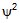gives an idea of intensity of electron wave i.e. probability of finding electron at that point.

Offer running on EduRev: Apply code STAYHOME200 to get INR 200 off on our premium plan EduRev Infinity!

## Chemistry Class 11

136 videos|245 docs|180 tests

,

,

,

,

,

,

,

,

,

,

,

,

,

,

,

,

,

,

,

,

,

;